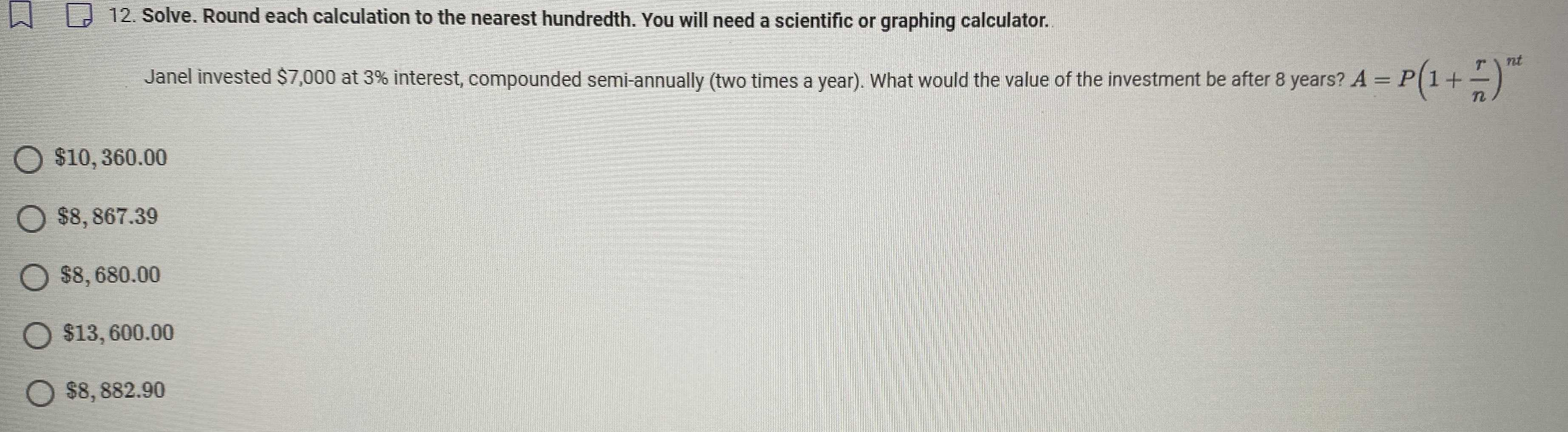### ¿Todavía tienes preguntas de matemáticas?

Pregunte a nuestros tutores expertos
Algebra
Pregunta$$\square 12$$ . Solve. Round each calculation to the nearest hundredth. You will need a scientific or graphing calculator.

Janel invested $$\ 7,000$$ at $$3 \%$$ interest, compounded semi-annually (two times a year). What would the value of the investment be after $$8$$ years? $$A = P ( 1 + \frac { r } { n } ) ^ { n t }$$

$$\ 10,360.00$$

$$\ 8,867.39$$

$$\ 8,680.00$$

$$\ 13,600.00$$

$$\ 8,882.90$$